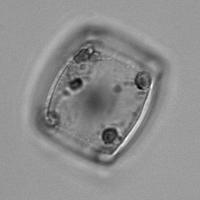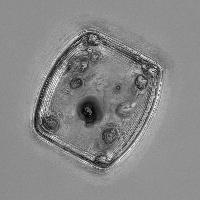# Tutorial: Extended Depth of Field¶

This is an example of how to use mahotas to implement an algorithm that it does not have built-in: extended depth of field.

The idea is that you have a stack of images, taken at different focal points, and you build a single image so that you get everything in focus.

```import numpy as np
import mahotas as mh
```

We are going to assume that you have an `image` object, which has three dimensions: the stack, height, and width:

```stack,h,w = image.shape
```

We use `mh.sobel` as the measure of “infocusness” for each pixel :

```focus = np.array([mh.sobel(t, just_filter=True) for t in image])
```

Now, we select the best slice at each pixel location:

```best = np.argmax(focus, 0)
```

So far, very easy. The next part is the hard part. We want to do the following:

```r = np.zeros((h,w))-1
for y in xrange(h):
for x in xrange(w):
r[y,x] = image[best[y,x], y, x]
```

But this is very slow (never run nested loops in Python if you can avoid it). We get the same result with a slightly less legible, but faster manipulation :

```image = image.reshape((stack,-1)) # image is now (stack, nr_pixels)
image = image.transpose() # image is now (nr_pixels, stack)
r = image[np.arange(len(image)), best.ravel()] # Select the right pixel at each location
r = r.reshape((h,w)) # reshape to get final result
```

Et voilà!

Here is an example, from a stack of microbes imaged. This is is the maximum intensity projection:This is the most in-focus slice (using the sobel operator as the measure):And this is the extended depth of field result:It is clearly sharper, perhaps at the expense of some possible noise. I actually played around with blurring the image a little bit and it did improve things ever so slightly.

  Other methods simply use a different measure here.
  I am not 100% convinced that this is the best. After all we create an array of size `len(image)` just to index. I would be happy to find an alternative.